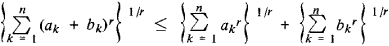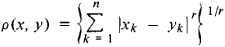# Minkowski Inequality

Also found in: Wikipedia.
The following article is from The Great Soviet Encyclopedia (1979). It might be outdated or ideologically biased.

## Minkowski Inequality

an inequality of the formwhere ak and ak (k = 1,2, . . . , n) are non-negative numbers and r > 1. The Minkowski inequality has analogs for infinite series and integrals. The inequality was established by H. Minkowski in 1896 and expresses the fact that in n-dimensional space, where the distance between the points x = (x1, x2, . . . , xn) and y = (y1, y2, . . . , yn) is given bythe sum of the lengths of two sides of a triangle is greater than the length of the third side.

Mentioned in ?
References in periodicals archive ?
By (31), the Minkowski inequality, and the generalized Holder inequality we have
From the "Minkowski inequality" (Proposition 4.6) we can obtain analogous estimates if we take the 2 in the last position and move it backwards making it take every position from the last to the first; in other words, considering the following exponents:
By the Holder inequality, Minkowski inequality combined with the estimate of (39), and Young inequality, we obtain
Varosanec, Properties of mappings related to the Minkowski inequality, Mediterranean J.
The purpose of this paper first is to establish the Minkowski inequality for the dual Quermassintegral sum, which is a generalization of the Minkowski inequality for mixed intersection bodies.
If t [greater than or equal to] 1, then by Minkowski inequality we obtain
It follows easily from Fubini-Tonnelli's theorem forp = 1, the generalized Minkowski inequality (see , p.
The new Orlicz-Minkowski and Brunn-Minkowski inequalities for the Orlicz mean dual affine quermassintegrals in special case yield the [L.sub.p]-dual Minkowski inequality and Brunn-Minkowski inequalities for the mean dual affine quermassintegrals, which also imply the dual Orlicz-Minkowski inequality and Brunn-Minkowski inequalities for general volumes.
Using the Minkowski inequality ([4, p.21]) for r > 1 and followed by the Holder inequality ([4, p.22]) with exponents s - t/r - t > 1 and s - t/s - r, we obtain
Proof From (12), (13), (21) and notice for i < n - 1 to use the Minkowski inequality for integral [14)P.147], we obtain for p [greater than or equal to] 1
Note first that by the Holder or Minkowski inequality, with [X.sub.m] and [Y.sub.m] defined by (2.2),
A Minkowski inequality for mixed quermassintegrals states that, for K,L [member of] [K.sup.n] and 0 [less than or equal to] i < n -1,

Site: Follow: Share:
Open / Close